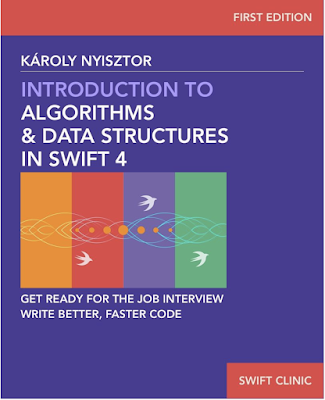Download Introduction to Algorithms and Data Structures in Swift 4 - Programming Ebook

# Programming Ebook

## Tuesday, June 5, 2018Introduction to Algorithms and Data Structures in Swift 4

Book Details
TitleIntroduction to Algorithms and Data Structures in Swift 4
ISBN-10978-1973291749
Author: Karoly Nyiszto
Language: English
SubjectSwift / Computers & Technology / Programming / Apple Programming
No. of pages: 247
FormatEPUB/MOBI/AZW3

Introduction

Thank you for buying my book “Introduction to Algorithms and Data Structures in Swift 4”. This book is going to teach you fundamental knowledge about algorithms and data structures.

What’s Covered In This Book?

First, we’ll talk about the Big-O notation, which is a mathematical model for measuring and classifying algorithms. It estimates the time efficiency of running an algorithm as a function of the input size.
We are going to talk about constant time, linear time, polynomial time, and logarithmic time. To understand these concepts, we’re going to implement, analyze and compare a various algorithms.

The next chapter is about recursion. Many algorithms and data structures rely on recursion. Thus, understanding how recursion works is imperative.

Next, I am going to show you the power of algorithms. We’ll compare simple, unoptimized samples with ones which rely on algorithms. If you had any doubts about the importance of algorithms, these examples are going to convince you.

Then, we’ll delve into generics. You must understand generics before we start studying data structures and algorithms.

Next, I’m going to walk you through the built-in Swift collection types.

Then we jump into the topic of basic sorting algorithms. We’ll talk about selection sort, bubble sort, and insertion sort. We’ll analyze the efficiency of each of these sorting algorithms, and we are going to represent them visually.

We’ll then talk about two, more advanced sorting algorithms: merge sort and quicksort.

Finally, I’m going to share with you some useful online resources which will help you in sharpening your coding and problem solving skills.

After finishing this book, you’ll know all the concepts related to algorithms and data structures. You’ll have a good understanding of how the popular search algorithms work and the way they can be implemented using Swift.

As the first part of a series on Swift programming, this book is an essential steppingstone for delving deeper into algorithms, data structures and programming in general.